Search by Topic

Resources tagged with Representing similar to Let Us Divide!:

Filter by: Content type:
Age range:
Challenge level:

There are 15 results

Broad Topics > Using, Applying and Reasoning about Mathematics > RepresentingLet Us Divide!

Age 7 to 11 Challenge Level:

Look at different ways of dividing things. What do they mean? How might you show them in a picture, with things, with numbers and symbols?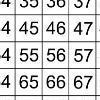That Number Square!

Age 5 to 11 Challenge Level:

Exploring the structure of a number square: how quickly can you put the number tiles in the right place on the grid?Different Sizes

Age 5 to 11 Challenge Level:

A simple visual exploration into halving and doubling.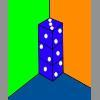Dice in a Corner

Age 7 to 11 Challenge Level:

How could you arrange at least two dice in a stack so that the total of the visible spots is 18?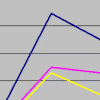Charting More Success

Age 11 to 16 Challenge Level:

Can you make sense of the charts and diagrams that are created and used by sports competitors, trainers and statisticians?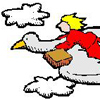A Flying Holiday

Age 7 to 11 Short Challenge Level:

Follow the journey taken by this bird and let us know for how long and in what direction it must fly to return to its starting point.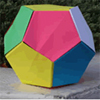Dodecamagic

Age 7 to 11 Challenge Level:

Here you see the front and back views of a dodecahedron. Each vertex has been numbered so that the numbers around each pentagonal face add up to 65. Can you find all the missing numbers?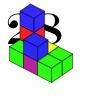28 and It's Upward and Onward

Age 7 to 11 Challenge Level:

Can you find ways of joining cubes together so that 28 faces are visible?Going for Gold

Age 7 to 11 Challenge Level:

Looking at the 2012 Olympic Medal table, can you see how the data is organised? Could the results be presented differently to give another nation the top place?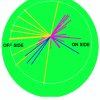Charting Success

Age 11 to 16 Challenge Level:

Can you make sense of the charts and diagrams that are created and used by sports competitors, trainers and statisticians?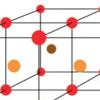Finding 3D Stacks

Age 7 to 11 Challenge Level:

Can you find a way of counting the spheres in these arrangements?3D Stacks

Age 7 to 14 Challenge Level:

Can you find a way of representing these arrangements of balls?Olympic Turns

Age 7 to 11 Challenge Level:

This task looks at the different turns involved in different Olympic sports as a way of exploring the mathematics of turns and angles.The Development of Algebra - 2

Age 11 to 18

This is the second article in a two part series on the history of Algebra from about 2000 BCE to about 1000 CE.What Is the Question?

Age 11 to 16 Challenge Level:

These pictures and answers leave the viewer with the problem "What is the Question". Can you give the question and how the answer follows?